Subsections

# 4.3 SkiplistList: An Efficient Random-Access List

A SkiplistList implements the List interface using a skiplist structure. In a SkiplistList,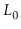contains the elements of the list in the order in which they appear in the list. As in a SkiplistSSet, elements can be added, removed, and accessed in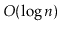time.

For this to be possible, we need a way to follow the search path for the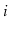th element in. The easiest way to do this is to define the notion of the length of an edge in some list,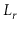. We define the length of every edge inas 1. The length of an edge,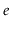, in,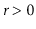, is defined as the sum of the lengths of the edges belowin. Equivalently, the length ofis the number of edges inbelow. See Figure 4.5 for an example of a skiplist with the lengths of its edges shown. Since the edges of skiplists are stored in arrays, the lengths can be stored the same way: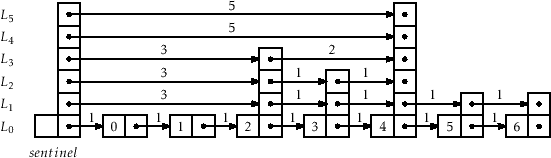The useful property of this definition of length is that, if we are currently at a node that is at position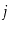inand we follow an edge of length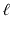, then we move to a node whose position, in, is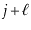. In this way, while following a search path, we can keep track of the position,, of the current node in. When at a node,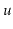, in, we go right ifplus the length of the edge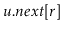is less than. Otherwise, we go down into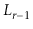.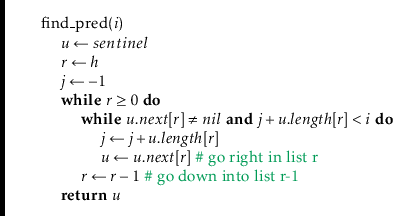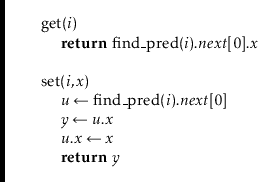Since the hardest part of the operations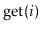and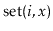is finding theth node in, these operations run intime.

Adding an element to a SkiplistList at a position,, is fairly simple. Unlike in a SkiplistSSet, we are sure that a new node will actually be added, so we can do the addition at the same time as we search for the new node's location. We first pick the height,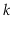, of the newly inserted node,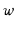, and then follow the search path for. Any time the search path moves down fromwith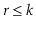, we spliceinto. The only extra care needed is to ensure that the lengths of edges are updated properly. See Figure 4.6.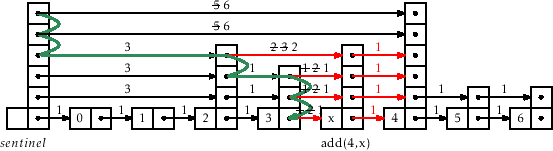Note that, each time the search path goes down at a node,, in, the length of the edgeincreases by one, since we are adding an element below that edge at position. Splicing the nodebetween two nodes,and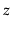, works as shown in Figure 4.7. While following the search path we are already keeping track of the position,, ofin. Therefore, we know that the length of the edge fromtois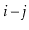. We can also deduce the length of the edge fromtofrom the length,, of the edge fromto. Therefore, we can splice inand update the lengths of the edges in constant time.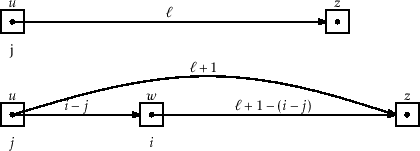This sounds more complicated than it is, for the code is actually quite simple: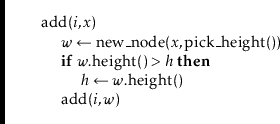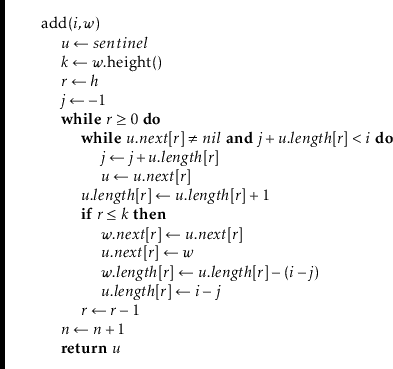By now, the implementation of the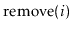operation in a SkiplistList should be obvious. We follow the search path for the node at position. Each time the search path takes a step down from a node,, at level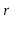we decrement the length of the edge leavingat that level. We also check ifis the element of rankand, if so, splice it out of the list at that level. An example is shown in Figure 4.8.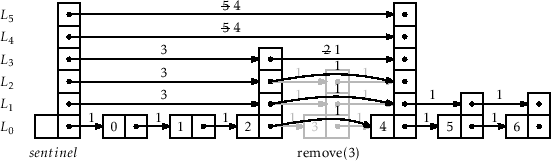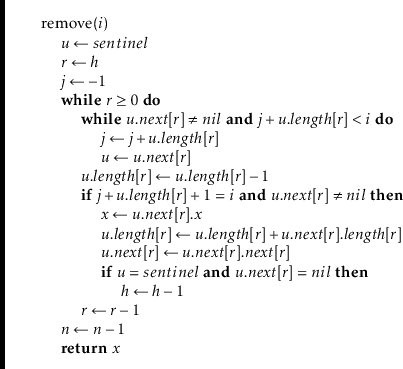## 4.3.1 Summary

The following theorem summarizes the performance of the SkiplistList data structure:

Theorem 4..2   A SkiplistList implements the List interface. A SkiplistList supports the operations,,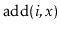, andinexpected time per operation.

opendatastructures.org